# Missing Characters - Solved Examples

Q 1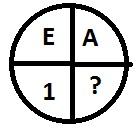Options :

A - 5

B - 2

C - 3

D - 4

Explanation

In alphabetic order of `A’ is one while that of E is 5.

Q 2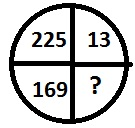Options :

A - 9

B - 11

C - 15

D - 12

Explanation

The numbers have their square in diagonally opposite space.

Q 3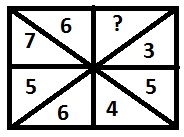Options :

A - 2

B - 9

C - 5

D - 8

Explanation

The numbers are changing in clockwise direction as by adding 2 and subtracting 1 alternately starting from 3. Hence missing number is 8.

Q 4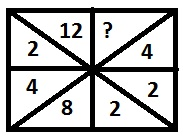Options :

A - 7

B - 9

C - 6

D - 8

Explanation

Half of the previous number is being placed in following space and 2 is getting place after each two steps.

Q 5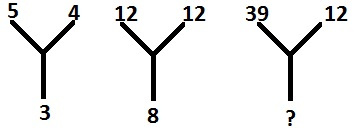Options :

A - 15

B - 17

C - 19

D - 21

Explanation

The number at the bottom is obtained as, $\frac{5+4}{3} = 3$ and $\frac{12+12}{3} = 8$, similarly $\frac{12+39}{3} = 17$. Therefore missing number is 17.

Q 6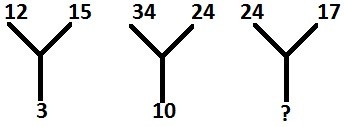Options :

A - 4

B - 9

C - 11

D - 7

Explanation

Numbers at bottom are the difference of numbers at the top, therefore missing number is 24 - 17 =7.

Q 7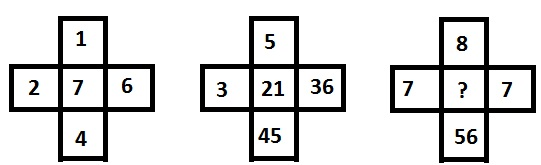Options :

A - 5

B - 7

C - 8

D - 12

Explanation

The numbers are obtained as $\frac{4}{1}+ \frac{6}{2} = 7$ and $\frac{45}{5} + \frac{36}{3} = 21$, similarly $\frac{7}{7}+ \frac{56}{8} = 8$.

Q 8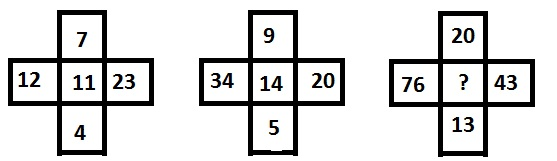Options :

A - 25

B - 14

C - 62

D - 33

Explanation

The numbers are related as 23 - 12 = 11 = 7+4 and 34 - 20 = 14 = 9 + 5, similarly 76 - 43 = 33 = 20 + 13.

Q 9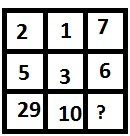Options :

A - 68

B - 89

C - 85

D - 90

Explanation

Numbers are obtained as, 22 + 52 = 29 and 12 +32 = 10, therefore missing number is 72 +62 = 85.

Q 10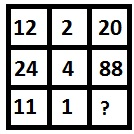Options :

A - 14

B - 18

C - 23

D - 12